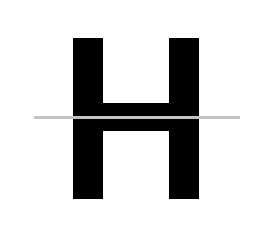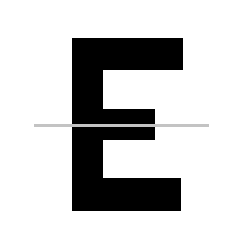Courses
Courses for Kids
Free study material
Offline Centres
MoreLast updated date: 07th Dec 2023
Total views: 280.2k
Views today: 2.80k

# Which of the following alphabet has the same horizontal mirror line image?A. HB. BC. ED. All the aboveVerified
280.2k+ views
Hint: Mirror line image is nothing but the symmetry line. Here they have given a horizontal mirror line, that is horizontal symmetry line. A line that cuts an image or an object into half where both halves are a mirror image of each other is called symmetry. We have two types of line of symmetry: one is the horizontal line of symmetry and the other one is the vertical line of symmetry.

The given alphabets are H, B and E. Let us first draw the horizontal mirror line, that is, horizontal line of symmetry.
Let us consider the first option (a) H. Draw the horizontal mirror line at the middle of the alphabet H.A we can see that after drawing the horizontal mirror line to the alphabet H, both the halves represent the mirror image of each other. Thus, the alphabet H has a mirror image when a horizontal line is drawn.
Now let’s see the second option (b) B. Draw a horizontal mirror line at the center of it.We can see that both the halves are the mirror image of each other. Thus, the alphabet B has a mirror image when a horizontal line is drawn.
Now let us consider the option (c) E. Draw the horizontal mirror line to the alphabet E.A we can see that after drawing the horizontal mirror line to the alphabet E, both the halves represent the mirror image of each other. Thus, the alphabet E has a mirror image when a horizontal line is drawn.
Since the three options (a) H, (b) B and (c) E have the horizontal mirror image, option (d) All the above is the correct answer for this question.
So, the correct answer is “Option D”.

Note: As we have seen that there are two types of lines of symmetry horizontal and vertical, since they have mentioned that horizontal mirror line, we have to use the horizontal line only. If they didn’t mention any specific line we can go for any line of symmetry, either horizontal or vertical.